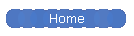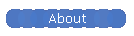How to obtain TV Video Output From an FPGA

This project arose from my desire to have color video output to a TV rather than an expensive monitor. Most of the sample circuits I've seen for hobbyist type video output use VGA output to drive a monitor. Interfacing to a VGA monitor is relatively easy and provides a high quality video output. But what do you do if you don't have a VGA monitor to spare ? Many hobby type projects do not have the memory capacity or performance to drive a VGA monitor.  Even used VGA monitors in good working order can be relatively expensive. How about using a TV instead ? It turns out that interfacing to a TV is almost as easy as interfacing to a VGA monitor. I came up with the following two circuits, the first of which I've built, the second of which I'm pretty sure will work. Schematics for the circuits are here: vdac.pcx

Descriptions:

Circuit A

Circuit A uses the commonly, widely available and inexpensive DAC08 D to A converter chip to convert an eight bit FPGA output to a signal voltage level. In order to get a D to A output with more than about five bits of precision requires using a DAC chip. Rather than explaining in detail how the DAC works I suggest reading the data sheets instead. Basically the DAC takes a reference current and multiplies it by the digital input divided by 256. This gives an output current range (which flows into the output pins) from 0 to 255/256 of the reference current. The output current from the DAC is converted into a voltage and used to drive a darlington transistor (Q1 and Q2) which acts as an impedance buffer for the TV output. The darlington transistor is configured as an emitter follower so the voltage at the emitter reflects the voltage at the base (minus 1.4V dropped from the base to emitter). The trick with this circuit is to bias the transistor properly and use the right DAC output. For the lowest output level (sync) we would like the output to be 0V which translates to 1.4V at the base of the transistor. For the maximum video output, the output signal should go to about 1V which translates to 2.4V at the base of the transistor. Using a voltage divider bias circuit a pair of resistors (R1 and R2) has to be chosen so that the voltage varies between 1.4V and 2.4V over the full scale range of the DAC. We make use of the fact that the DAC acts like a load in parallel with R2. The DAC uses a 0.5mA reference current, so at maximum output 0.5mA of current flows into the DAC. With the DAC connected to the base of the transistor, the lowest voltage will be present when the DAC draws maximum current as this will result in the greatest voltage drop across R1. When the DAC draws no current this will result in the highest voltage at the base of the transistor, the voltage being determined solely by the R1/R2 voltage divider. So, the DAC's complement output is used to obtain this behaviour. We have R1 / (R1+R2) * 5V = 2.4V and  (I + Iref) * R1 = 3.6V. All of this boils down to resistor values of 3.6k and 3.3k for R1 and R2 respectively.

Using an eight bit D/A converter chip is sufficient (in theory) to get the same number of colors as a fifteen bit VGA output due to the color encoding mechanism. Effectively five bit RGB values are summed (5bits+5bits+5bits) producing a maximum seven bit sum. You don't get something for nothing and what is lost is the horizontal resolution for color information.

Also note, the DAC has a settling time of about 80ns. This means it's not really useful beyond 8 or 10 MHz although I have tried an 80 column monochrome display (14MHz) on the TV and it is readable.

Circuit B

Some systems already have a 15 or 16 bit VGA output built using simple resistor DACs. It's possible to hijack the green signal output and add a transistor buffer and resistor to obtain a TV compatible output. Note in this case the transistor has to be directly driven from the VGA signal output as any voltage biasing network would affect the proper operation of the resistor DAC. (The transistor buffer itself has a high enough resistance that it shouldn't load the DAC). Because of the fact that the transistor is directly DC coupled to the DAC network, the full range of the DAC isn't available for use in the TV signal. The darlington transistor needs to see about 1.4V before it will turn on, and the TV video signal should also be limited to about 1V. This means only the DAC output range from 1.4 to 2.4V is usable, which is about one third of the range of the DAC. Which means that about two bits worth of the value of the DAC are lost, making the effective usefulness of the DAC three bits.

Note that you can't use this circuit with the VGA monitor connected as the VGA load resistance will limit the output to about 1V which isn't enough to turn on the transistor.

Circuit C